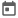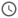# Array Manipulations in System Verilog##### 10 Apr 2022##### 4 mins

Arrays as we have seen are used to store lots of data in one entity. At times we would require performing various operations on the elements stored, like searching a particular element, sorting the array in an order, finding sum of all the elements present in array, etc. System Verilog provides many inbuilt functions with the help of which we can perform various types of array manipulation or operations on array. Before looking into the methods lets discuss about the `with` keyword is a important to pass conditions.

### with keyword

Basically, `with` keyword is used to pass a condition. This keyword is used with a function to put some constraint for the argument which we are passing as the function. These keywords are not only used with the array manipulation functions but also with some other constructs which we will see later in this series.

Syntax – `function_name([argument]) with ([argument condition]);`

We will see the exact working of the with keyword in below examples.

### Array ordering functions

These function changes the order of the elements present in array. They can be used to sort the array in descending or ascending array or shuffle the arrays.

1. reverse() – This function reverses the order of the array. This means that the first element will be shifted to the last position and last element will come to the first position.
2. sort() – This function sorts the array in the ascending order. This can be used in conjunction with the `with` keyword.
3. rsort() – This function sorts the array in descending order. This also supports the `with` keyword optionally.
4. shuffle() – Randomly changes the order of the element present in array.
We cannot use the with keyword with reverse and shuffle functions.

#### Example

``````module ordering_example();
int arr[] = '{12, 45, 23, 89, 23, 43, 23, 78, 98};
int res[];

initial begin
arr.reverse();
\$display("arr.reverse() = %p", arr);

arr.sort() with (item > 40);
\$display("arr.sort() + with = %p", arr);

arr.sort();
\$display("arr.sort() = %p", arr);

arr.rsort();
\$display("arr.rsort() = %p", arr);

arr.shuffle();
\$display("arr.shuffle() = %p", arr);
end
endmodule``````
##### Output
``````# arr.reverse() = '{98, 78, 23, 43, 23, 89, 23, 45, 12}
# arr.sort() + with = '{23, 23, 23, 12, 89, 78, 45, 98, 43}
# arr.sort() = '{12, 23, 23, 23, 43, 45, 78, 89, 98}
# arr.rsort() = '{98, 89, 78, 45, 43, 23, 23, 23, 12}
# arr.shuffle() = '{23, 89, 98, 78, 23, 45, 43, 23, 12}``````

### Array searching functions

These functions help us to find certain elements of the array based on some condition or value of the elements. Also, we can use these functions to find the min or max value present in the array. Let’s explore different functions in detail:

#### find functions

1. find() – This function returns all the elements which satisfy a given condition.
2. find_index() – Returns the index of all the elements which satisfy a given condition. This is somewhat similar to find() but returns the index instead of actual elements.
3. find_first() – returns the first element which satisfies the given condition.
4. find_first_index() – returns the index of the first element which satisfies the given condition.
5. find_last – returns the last element which satisfies the given condition.
6. find_last_index() – returns the index of the last element which satisfies the given condition.
`with` clause is important with all these functions.
##### Example
``````module find_example();
int arr[] = '{12, 45, 23, 89, 1, 43, 65, 78, 98, 34, 54, 16, 28};
int res[];

initial begin
// This will print all the elements which are
// greater than 20
res = arr.find(x) with (x > 20); // here x is explicit iterator
\$display("res = %p", res);

res = arr.find() with (item > 40); // item is a implicit iterator
\$display("res = %p", res);

// This will print the index of all elements which are
// greater than 20
res = arr.find_index(x) with (x > 20);
\$display("res = %p", res);

// This will print the first element which is larger than 20
res = arr.find_first(x) with (x > 20);
\$display("res = %p", res);

// This will print the index of first element which is larger than 20
res = arr.find_last(x) with (x > 40);
\$display("res = %p", res);
end
endmodule``````
##### Output
``````# res = '{45, 23, 89, 43, 65, 78, 98, 34, 54, 28}
# res = '{45, 89, 43, 65, 78, 98, 54}
# res = '{1, 2, 3, 5, 6, 7, 8, 9, 10, 12}
# res = '{45}
# res = '{54}``````

#### Other search functions

1. min() – Returns the smallest value of the array
2. max() – Returns the largest value of the array
3. unique() – Returns the array in which all the duplicate values are removed.
4. unique_index() – Return the array of indices which contains unique value.
##### Example
``````module other_search_example();
int arr[] = '{12, 45, 23, 89, 23, 43, 23, 78, 98};
int res[];

initial begin
res = arr.min();
\$display("arr.min() = %p", res);

res = arr.max();
\$display("arr.max() = %p", res);

res = arr.unique();
\$display("arr.unique() = %p", res);

res = arr.unique_index();
\$display("arr.unique_index() = %p", res);
end
endmodule``````
##### Output
``````# arr.min() = '{12}
# arr.max() = '{98}
# arr.unique() = '{12, 23, 43, 45, 78, 89, 98}
# arr.unique_index() = '{0, 2, 5, 1, 7, 3, 8}``````

### Array reduction functions

These are special functions which can reduce the array into one element. In simple terms, these functions which perform certain operations on the elements on the array and return one element. For example, sum of all the elements is a type of reduction operation which gives one value as output.

1. sum() – Returns the sum of all array elements
2. product() – Returns the product of all array elements
3. and() – Returns the bitwise AND (&) of all array elements
4. or() – Returns the bitwise OR (|) of all array elements
5. xor() – Returns the bitwise XOR (^) of all array elements
Quote – In reduction function the output will depend on the width of the data type used for array. For example if arrays is of `bit[5:0]` data type, the output will also be of `bit[5:0]`. Any extra bits will be ignored in the output.

#### Example

``````module reduction_example();
bit[5:0] arr[] = '{12, 45, 23};
int res;
bit[5:0] red_res;

initial begin
res = arr.sum();
\$display("arr.sum() = %0d", res);

res = arr.product();
\$display("arr.product() = %0d\n", res);

red_res = arr.and();
\$displayb("arr = %p", arr);
\$display("arr.and() = %b", red_res);

red_res = arr.or();
\$display("arr.or() = %b", red_res);

red_res = arr.xor();
\$display("arr.xor() = %b", red_res);
end
endmodule``````
##### Output
``````# arr.sum() = 16
# arr.product() = 4
#
# arr = '{001100, 101101, 010111}
# arr.and() = 000100
# arr.or() = 111111
# arr.xor() = 110110``````
Try the examples in eda playground